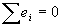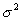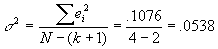Economics 345 Midterm

Prof. Bryan Caplan

Fall, 1997

Mean: 52.14 SD: 16.97 Min: 15 Max: 79

Part 1: True, False, and Explain

(10 points each - 3 for the right answer, and 7 for the explanation)

State whether each of the following six propositions is true or false. Using 2-3 sentences AND/OR equations, explain your answer.

1. The correlation coefficient between a constant and a random variable ranges between -1 and +1.

FALSE. The correlation coefficient between a constant and a random variable is ALWAYS ZERO.

1. If, then the sum of squared errors will also equal zero.

FALSE. This is only true if the error terms are all exactly equal to zero.

#3 AND #4 refer to the following regression:

 Y XYi=1.237 + .847*Xi (.301) (.117) 4 3 3.778 4 3.5 4.216 3 2 2.931 2 1 2.084

3. The best estimate of the variance of the error termsis .0269.

FALSE.4. The null hypothesis that the coefficient on X is zero may be rejected at the 1% level.

TRUE. The critical value for 3 degrees of freedom is 5.841. .847± 5.841*.117= .847± 683, so you can reject the hypothesis that the coefficient on X is zero.

5. If you run the regression Wagei=a + b1*Experiencei + b2*(Experiencei)2, you would expect b1 to be positive and b2 to be negative.

TRUE. As discussed in class, you would expect this if the return to experience increases at a decreasing rate (and it does!).

1. Suppose you regress 1000 people's incomes on their experience, education, number of kids, and WOMAN (which =1 if the person is female, and 0 otherwise). Your results (in dollars) and SEs are as follows:

Income = 300 + 1200 *experience + 600 *education - 2000 *kids + 200 *WOMAN

(200) (100) (100) (300) (300)

These results show that on average, the income of women is not different from that of men in a statistically significant way.

FALSE. The income of women may be very different from that of men. This equation just shows that controlling for other factors women don't make less money, not that they don't in fact make less money.

(20 points each)

In 4-6 sentences AND/OR equations, answer both of the following questions.

1. Using HUMMER and your data on Nominal GDP and M2, you run two regressions:

DEFINE NGDPPER=((NGDP/LAG(NGDP)-1)*100

DEFINE M2PER=((M2/LAG(M2)-1)*100

DEFINE MRAT=M2/LAG(M2)

REGRESS NGDPPER ON M2PER

REGRESS NGDPPER ON MRAT

If the results for the first regression are NGDPPER = 1 + .9 * M2PER, can you figure out what the results for the second regression are? If so, what are the results? If not, why not? (Hint: Remember the rules of linear transformations.)

By the rules of linear transformations, dividing a variable by 100 makes the coefficient on that variable increase by a factor of 100. So if the first regression had b=.9, then b for the second regression will be 90. Similarly, adding 1 to a regression reduces a by 1*b, so a falls by 90. The equation for the second regression will therefore be:

NGDPPER = -89 + 90 * MRAT

2. TRAINING is the number of years a person has spent in worker training programs. If you regress INCOME on TRAINING, you will often find a negative impact of TRAINING on INCOME. Discuss why this may be the result of omitted variable bias, and suggest TWO variables you might add to the regression equation to get a better estimate of the true impact of TRAINING.

This could be the result of omitted variable bias because the workers in a worker training program may not be typical of other workers. You could control for years spent in prison and IQ to get better results.